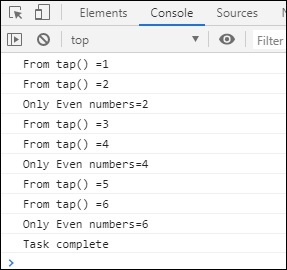# RxJS - Utility Operator tap

This operator will have the output the same as the source observable and can be used to log the values to the user from the observable. The main value, error if any or is the task is complete.

## Syntax

```tap(observer, error, complete):Observable
```

## Parameters

observer − (optional) this is the same asas source observable.

error − (optional) error method if any error occurs.

complete − (optional) complete() method will get called when the task is complete.

## Return value

It returns an observable same like source observable with a callback function.

## Example

```import { of } from 'rxjs';
import { tap, filter } from 'rxjs/operators';

let list1 = of(1, 2, 3, 4, 5, 6);
let final_val = list1.pipe(
tap(x => console.log("From tap() =" + x),
e => console.log(e),
filter(a => a % 2 === 0)
);
final_val.subscribe(x => console.log("Only Even numbers=" + x));
```

## Output